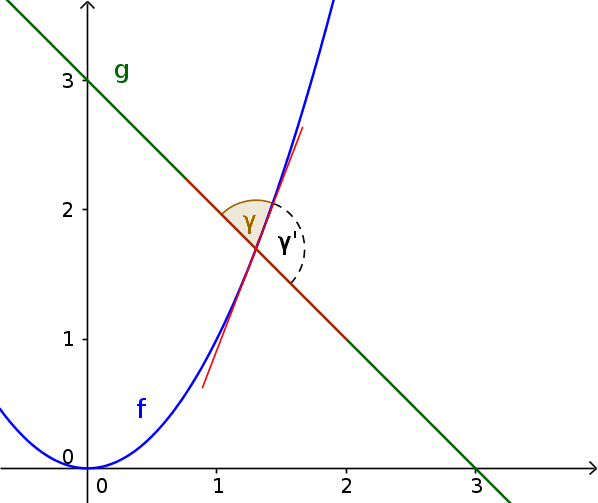Math Derivative applications Angle of intersection

# Angle of intersection

When the graphs intersect with two functions, the tangents form two angles with each other. The smaller angle is called the angle of intersection.The picture: By cutting the two tangents (red) two angles are formed: $\gamma$ and $\gamma'$.
$\gamma$ is the angle of intersection.

!

### Remember

$f$ and $g$ intersect at point $x$.

First, you need the slope angles of the functions:
$\alpha=\arctan(f'(x))$
$\beta=\arctan(g'(x))$

The angle of intersection has the smaller value:
$|\alpha-\beta|$ or
$180^\circ-|\alpha-\beta|$
i

### Method

1. Take derivatives
2. Calculate slopes
3. Calculate slope angle
4. Specify the angle of intersection

### Example

What is the angle of intersection of the functions $f(x)=x^2$ and $g(x)=x+2$ at the intersection $P(2|4)$.

1. #### Take derivatives

$f(x)=x^2$
$f'(x)=2x$

$g(x)=x+2$
$g'(x)=1$
2. #### Calculate slopes

The slope of the two functions at the intersection is calculated ($x=2$).
$f'(2)=2\cdot2=4$
$g'(2)=1$
3. #### Calculate slope angle

$\alpha=\arctan(f'(x))$
$\alpha=\arctan(4)\approx75.96°$

$\beta=\arctan(g'(x))$
$\beta=\arctan(1)=45°$
4. #### Specify the angle of intersection

$\gamma_1=|\alpha-\beta|$
$\gamma_1=|75.96°-45°|$ $=30.96°$

$\gamma_2=180°-|\alpha-\beta|$
$\gamma_2=180°-30.96°$ $=149.04°$

$\gamma_1<\gamma_2$
=> The angle of intersection $\gamma$ is $30.96°$